# Class 10 Science: CBSE Sample Question Paper- Term II (2021-22) - 3 Notes | Study CBSE Sample Papers For Class 10 - Class 10

## Class 10: Class 10 Science: CBSE Sample Question Paper- Term II (2021-22) - 3 Notes | Study CBSE Sample Papers For Class 10 - Class 10

The document Class 10 Science: CBSE Sample Question Paper- Term II (2021-22) - 3 Notes | Study CBSE Sample Papers For Class 10 - Class 10 is a part of the Class 10 Course CBSE Sample Papers For Class 10.
All you need of Class 10 at this link: Class 10

Class-X

Time: 120 Minutes
M.M: 40

General Instructions:

1. All questions are compulsory.
2. The question paper has three sections and 15 questions.
3. Section–A has 7 questions of 2 marks each; Section–B has 6 questions of 3 marks each; and Section–C has 2 case based questions of 4 marks each.
4. Internal choices have been provided in some questions. A student has to attempt only one of the alternatives in such questions.
Section - A

Q.1. The molecule of alkene family is represented by the general formula CnH2n. Now answer the following questions:
(a) What do n and 2n signify?
(b) What is the IUPAC name of alkene when n = 4?

(a) In general formula CnH2n, n indicates the number of carbon atoms and 2n indicates the number of hydrogen atoms present in the molecule.

(b) C4H8, Butene.

Q.2. The atomic number of three elements are given below: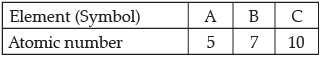Write the symbol of the element which belongs to (a) group 13, (b) group 15, of the periodic table. State the period of the periodic table to which these elements belong. Give reason to support your answer.

(a) A belongs to group 13 because its electronic configuration is 2, 3, i.e., it has 3 valence electrons.
(b) ‘B’ belongs to group 15 because its electronic configuration is 2, 5, i.e., it has 5 valence electrons.
They belong to the 2nd period as they both have two shells.

Q.3. The picture given below depicts the process of asexual reproduction in Plasmodium.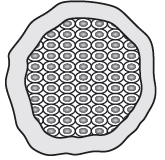(a) Name the process depicted above and define it.
(b) What is meant by asexual reproduction?

(a) Multiple Fission. It is a division of single celled organisms into many daughter cells simultaneously.
(b) Creation of a new generation from a single individual or a single parent is known as asexual reproduction.

Q.4. A student noticed that an organism by mistake was cut into two parts. After sometimes, he noticed that both the parts developed into new individuals.
(a) Name the mode of reproduction used by the organism. State an example of such organism which can multiply by this process.
(b) State the type of cells which can carry out this process.

(a) The mode of reproduction used by the organism is regeneration. Hydra or Planaria. (any one example)
(b) It is carried out by the specialized cells which can proliferate and make a large number of cells by cell division.

Q.5. Different species use different strategies to determine sex of a newborn individual. It can be determined by environmental cues or can be genetically determined.
Explain the statement by giving examples for each strategy.
OR
In human beings, the statistical probability of getting either a male or a female child is 50%. Give reasons.

Environmental Cue:
(i)
In some animals, the temperature at which fertilized eggs are kept determines whether the developing animal in the egg is male or female.
(ii) In some animals like snail, individuals can change sex.
Genetic Cue: A child who inherits an X-chromosome from the father will be a girl and one who inherits a Y-chromosome from the father will be a boy.
OR
A child who inherits an "X" chromosome from the father would be a girl (XX) while a child who inherits a "Y". chromosome from the father would be a boy (XY).
Since, the sex of the child is determined by what he/ she inherit from father, therefore the probability of getting either male or female child is 50%.

Q.6. Diagram shows lengthwise two sections of a current carrying solenoid. Ä indicates current entering into the page, and e indicates current emerging out of the page.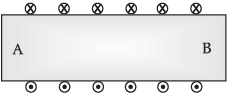(a) Decide which end of the solenoid A or B will behave as the north pole. Give reason for your answer.
(b) Also draw the field lines inside the solenoid.
OR
A coil of insulated copper wire is connected to a galvanometer as shown in the diagram. State your observations when a bar magnet is: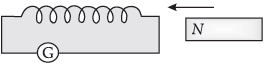(a) Pushed into the coil with its north pole entering first.
(b) Held at rest inside the coil.

(a) A = North Pole, B = South Pole.
Watching from one end of the solenoid if the current flows anti-clockwise, then North pole is developed at that end and if the current flows clockwise, then South pole is developed at that end.
(b)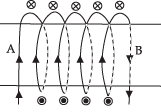OR
(a) If a bar magnet is pushed into the coil, an electric current will be induced in the coil due to electromagnetic induction. Therefore, the galvanometer will show some deflection.
(b) If a bar magnet is held stationary inside the coil, then no current is induced and therefore there is no deflection in the galvanometer.

Q.7. In a biology class, teacher taught students about the energy flow by drawing a food chain. In the following food chain, plants provide 5000 J of energy to grasshopper. How much energy will be available to owl from snakes? Can the organisms of any trophic level be removed?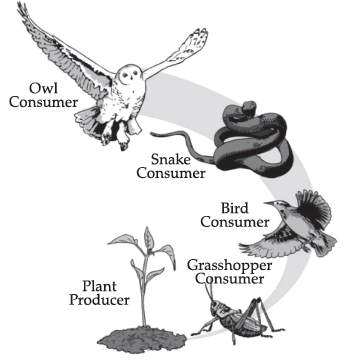OR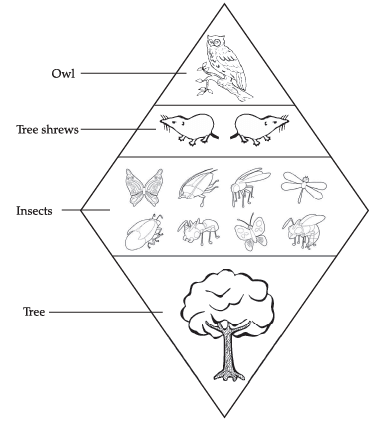Describe the flow of energy to the owl, if the tree provides 1500 calories of energy to the insects.

Energy available to owl is 5 J. Energy available  to grasshopper = 5000 J.
Bird = (5000 x 10)/100 = 500 J.
Snake = 500 x (10/100) ⇒ 50J.
Owl = 50 x (10/100) = 5 J
(According to 10 percent Law)
Removal of organisms at a trophic level will upset the whole ecosystem as all categories of organisms are linked through a food chain. The survival of organisms of one trophic level depends on the existence of the members of another trophic level.
OR
The insects would provide 10 percent (one tenth) of 1500 calories, or 150 calories, of energy to the shrews.
The shrews would provide one tenth of 150 calories, or 15 calories, to the owl.

Section - B

Q.8. Choose an element from period 3 of modern periodic table that matches the description given below in each instance. Give reason for your choice.
(a) It has a similar structure to diamond.
(b) It has same valency as Lithium.
(c) It has variable valency and is a member of the Oxygen family (group 16).

(a) Silicon
Reason: Tetrahedral structure
OR
Tetravalency or four valeny and catenation
OR
Covalent bonding like carbon
(b) Sodium
Reason: It has 1 valence electron like Lithium
(c) Sulphur
Reason: It forms oxides SO2 and SO3

Q.9. (a) How many isomers are possible for the compound with the molecular formula C4H8? Draw electron dot structure of branched chain isomer.
(b) How will you prove that C4H8 and C5H10 are homologous?
OR
A carbon compound ‘A’ having melting point 156K and boiling point 351K, with molecular formula C2H6O is soluble in water in all proportions.
(a) Identify ‘A’ and draw its electron dot structure.
(b) Give the molecular formulae of any two homologous of ‘A’.

(a) Five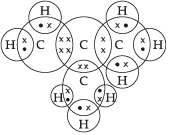(b) C4H8 and C5H10 are homologous as they differ in

• “–CH2–”
• differ in 14u molecular mass
• Same functional group
• Same general formula

OR

(a) Ethanol; C2H5OH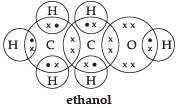(b) CH3OH and C3H7OH are homologous of ethanol.
OR
CH4O and C3H8O

Q.10. Two pea plants-one with round yellow (RRYY) seeds and another with wrinkled green (rryy) seeds produce F1 progeny that have round, yellow (RrYy) seeds.
When F1 plants are self-pollinated, which new combination of characters is expected in F2 progeny?
How many seeds with these new combinations of characters will be produced when a total of 160 seeds are produced in F2 generation? Explain with reason.

Round green: 30
Wrinkled yellow: 30
New combinations are produced because of the independent inheritance of seed shape and seed colour trait.

Q.11. (a) It would cost a man ₹3.50 to buy 1.0 kW h of electrical energy from the Main Electricity Board. His generator has a maximum power of 2.0 kW. The generator produces energy at this maximum power for 3 hours. Calculate how much it would cost to buy the same amount of energy from the Main Electricity Board.
(b) A student boils water in an electric kettle for 20 minutes. Using the same mains supply, he wants to reduce the boiling time of water. To do so, should he increase or decrease the length of the heating element? Justify your answer.

(a) E = P × T
So, E = 3 × 2 = 6 kWh
Cost of buying electricity from the main electricity board = 6 x 3.50 = ₹21.0
(b) To reduce the boiling time using the same mains supply, the rate of heat production should be large. We know that P = V2/R. Since V is constant, R should be decreased. Since R is directly proportional to L so, length should be decreased.

Q.12.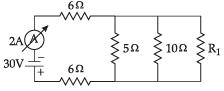In the above circuit, if the current reading in the ammeter A is 2A, what would be the value of R1?
OR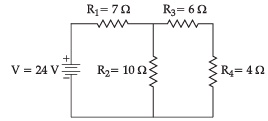Calculate the total resistance of the circuit and find the total current in the circuit.

5 ohm, 10 ohm and R1 are in parallel connection
1/Rp = 1/5 + 1/10 + 1/R1
1/Rp = (2+1)/10 + 1/R1
= 3/10 + 1/R1
1/Rp = (3R1 + 10)/10R1
R= 10R1/(3R1 + 10)
Now, 6 ohm, 6 ohm and Rp are in series
Thus,
Req = 12 + 10 R1/(3R1 + 10) ....(i)
V = I Req
From the circuit
Req = 30/2 = 15 Ω ...(ii)
Equating (i) and (ii)
12 + 10 R1/(3R1 + 10) = 15
10R1/(3R1 + 10) = 3
10R1 = (9R1 + 30)
Thus, R1 = 30 ohm.
OR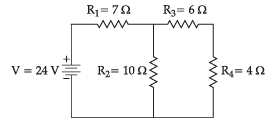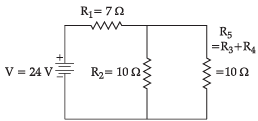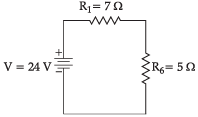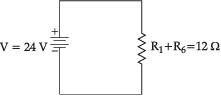R3 and Rare in series, hence the equivalent resistance of those two = R5 = R3 + R= 10 ohm.
R5 and R2 are in parallel, Let R6 be the equivalent resistance for them. Hence, R6 = (R5R2)/(R5 + R2) = 100/20 = 5 ohm
Now R1 and R6 are in series and hence the final equivalent resistance of the entire circuit is R = R1 + R6 = 12 ohm.
By Ohm's Law, we know that V = IR, hence I = V/R.
Hence the current in the circuit is 24/12 A = 2A

Q.13. Gas A, found in the upper layers of the atmosphere, is a deadly poison but is essential for all living beings. The amount of this gas started declining sharply in the 1980's.
(a) Identify Gas A. How is it formed at higher levels of the atmosphere?
(b) Why is it essential for all living beings? State the cause for the depletion of this gas.

(a) Gas A is Ozone.
Ozone at the higher levels of the atmosphere is a product of UV radiation acting on oxygen (O2) molecule. The higher energy UV radiations split apart some molecular oxygen (O2) into free oxygen (O) atoms. These atoms then combine with molecular oxygen to form ozone.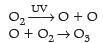(b) Ozone shields the surface of the earth and protects living organisms from ultraviolet (UV) radiations released by the sun.
Chlorofluorocarbons (CFC's) which are used as refrigerants in fire extinguishers lead to depletion of ozone layer.

Section - C

This section has 02 case-based questions (14 and 15). Each case is followed by 03 sub-questions (a), (b) and (c). Parts (a) and (b) are compulsory. However, an internal choice has been provided in part (c).

Q.14. If we cross-bred tall (dominant) pea plant with pure-bred dwarf (recessive) pea plant, we will get plants of F1 generation. Now, if we self-cross the pea plant of F1 generation, we obtain pea plants of F2 generation. Based on the given information, answer the following questions:
(a) What do the plants of F1 generation look like?
(b) State the ratio of tall plant to dwarf plants in F2 generation.
(c) State the type of plants not found in F1 generation but appeared in F2 generation. Write the reason for the same.
OR
Who is regarded as the ‘Father of Genetics’? Name the plant on which he performed his experiment.

(a) Tall (Tt, Tt, Tt, Tt)
(b) 3: 1 (TT, Tt, Tt and tt)
(c) Dwarf
Reason: Being a recessive trait, dwarfness can only be expressed in the recessive homozygous condition or in the absence of dominant trait.
OR
Gregor Johann Mendel, garden pea.

Q.15. Aashi observed the magnetic field lines of two bar magnets A and B as shown below.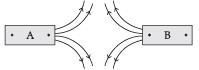(a) Name the poles of the magnets facing each other.
(b) How does the strength of the magnetic field at the centre of a current carrying circular coil depend on the:
(ii) Number of turns in the coil, and
(iii) Strength of the current flowing in the coil ?
(c) Two magnetic field lines never intersect each other. Why ?
OR
Why are magnetic field lines more crowded towards the pole of a magnet ?

(a) North poles.
(b) (i) Inversely proportional; larger the radius, weaker will magnetic field.
(ii) Directly proportional; more turns, more strong magnetic field.
(iii)Directly proportional; more strength of current, more strong magnetic field.
(c) The magnetic field lines never intersect each other because if two lines intersect each other, then at that point of intersection, the magnetic field has two directions, which is not possible.
OR
The magnetic field is stronger at the poles so the magnetic field lines are crowded at the poles.

The document Class 10 Science: CBSE Sample Question Paper- Term II (2021-22) - 3 Notes | Study CBSE Sample Papers For Class 10 - Class 10 is a part of the Class 10 Course CBSE Sample Papers For Class 10.
All you need of Class 10 at this link: Class 10Use Code STAYHOME200 and get INR 200 additional OFF

## CBSE Sample Papers For Class 10

174 docs|7 tests

Track your progress, build streaks, highlight & save important lessons and more!

,

,

,

,

,

,

,

,

,

,

,

,

,

,

,

,

,

,

,

,

,

;